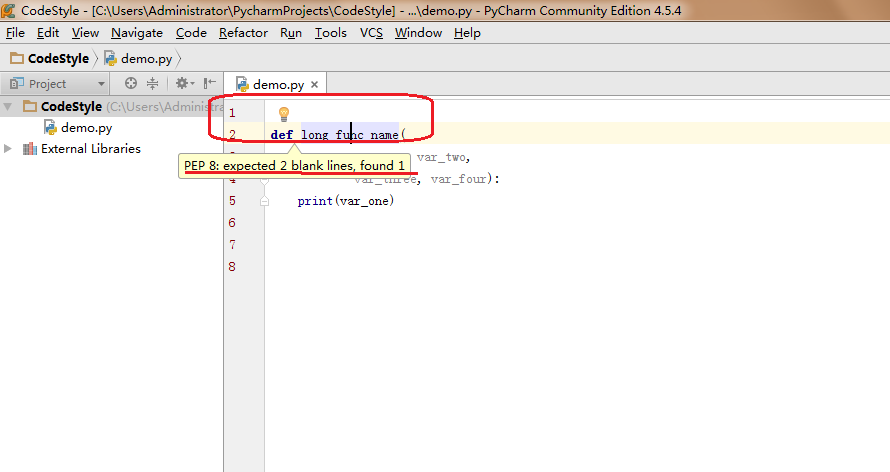### PEP8——Python代码规范

PyCharm这一Python IDE使用的正是著名的PEP8代码规范，我们会看到，当有不符合规范的代码出现时，编译器会以灰色下划波浪线给出相关提示，本文即告诉你如何写出没有灰色警告线的至少看上去很美的代码样式。# 缩进（indentation）

Yes

``````# 使用开分隔符（opening delimiter）进行对齐

foo = long_func_name(var_one, var_two,
var_three, var_four)

# 使用更多的缩进以和其他的代码单元区别开来
# 如下例的，参数部分比函数体多四个缩进以和函数体进行区别
def long_func_name(
var_one, var_two, var_three,
var_four)
print(var_one)

# 悬挂缩进（hanging indents）：增加一个缩进级别
foo = long_func_name(
var_one, var_two,
var_three, var_four)
# 或者：
foo = long_func_name(
var_one, var_two,
var_three, var_four
)

a = [1, 2, 3,
4, 5, 6]
a = [
1, 2, 3,
4, 5, 6
]
``````

No

``````# 当不适用垂直对齐时，禁止在第一行使用参数
# 换句话说，在垂直对齐时，才可在第一行使用参数
foo = lone_func_name(var_one, var_two,
var_three, var_four)

# 当缩进不足以区分代码结构时，增加一个缩进级别
def long_func_name(
var_one, var_two, var_three
var_four):
print(var_one)
``````

# 最大行长度

``````with open('') as file_1, \
open('') as file_2:

``````class Rectangle(Shape):
def __init__(self, width, height,
color='black', emphasis=None, highlight=0):
if (width == 0 and height == 0 and
color == 'red' and emphasis == 'strong' or
height > 100):
raise ValueError("sorry, you lose")
if width == 0 and height == 0 and (color == 'red' or emphasis is None):
raise ValueError("I don't think so -- values are %s, %s" %
(width, height))
Shape.__init__(self, width, height, color,
emphasis, highlight)``````

# 空行

• 顶级函数（当前文件中的第一个函数）或者顶级类（当前文件的第一个类）之前要有两个空行

• 定义在类内部的函数（成员函数）之间要留有一个空行

• 可以使用额外的空行（但要注意节制）以区分不同的函数组，

• 在一堆只有一行的函数之间不要使用空行（比如一些函数的空实现）

• 在函数内部使用空行，来标识不同的逻辑单元

# imports

• 在独立的行中导入不同的包

Yes

``````import sys
import os``````

No

``import sys, os``

``from subprocess import Popen, PIPE``
• import文件应当总是位于文件的首部，仅在模块备注和文档之后，在模块的全局变量和常量之前的位置

import文件的顺序：
1. 标准库（如sys、os）
2. 相关的第三方的库（如numpy、pandas、matplotlib）
3. 自定义的.py文件或者自定义的库

• 推荐使用绝对路径包含，因为可读性更好，并且不易出错。
``````import mypkg.sibling
from mypkg import sibling
from mypkg.sibling import example``````

``````from . import sibling
from .sibling import example        # .表示当前路径``````

• 应当避免通配符导入文件

# 表达式中的空格

• 紧跟着大括号、中括号和小括号之前

Yes

``spam(ham, {eggs: 90})``

No

``spam( ham [ 1 ], { eggs: 2 })``
• 紧连着逗号、分号、冒号之前

Yes

``if x == 4: print x, y; x, y = y, x``

NO

``if x == 4 : print x , y ; x , y = y , x ``
• 切片中的冒号

Yes

``````ham[1:9], ham[1:9:3], ham[:9:3], ham[1::3], ham[1:9:]
ham[lower:upper], ham[lower:upper:], ham[lower::step]
ham[lower+offset : upper+offset]
ham[: upper_fn(x) : step_fn(x)], ham[:: step_fn(x)]
ham[lower + offset : upper + offset]``````

No

``````ham[lower + offset:upper + offset]
ham[1: 9], ham[1 :9], ham[1:9 :3]
ham[lower : : upper]
ham[ : upper]``````

# 其他的建议

• 总是在如下的二元操作符的两边使用单空格：

1. 赋值：`=`
2. 增量赋值：`+=``-=`
3. 比较：`==`, `<`, `>`, `!=`, `<>`, `in` , `is`
4. 布尔：`and``or``not`
• 不要使用空格，当被用来标识一个关键字参数（使用函数）或者一个默认参数赋值（定义函数）

Yes

``````def complex(real, imag=0.):
return magic(r=real, i=imag)``````

No

``````def complex(real, imag = 0.):
return magic(r = real, i = imag)``````

# 文档

• 为所有公共模块或者函数、类以及方法编写文档。不必为非公共方法编写doc文档，但应有一个注释描述算法的功能，这条注释应当出现在`def`之后

• 结尾的`"""`应当独占一行

``````"""Return a foobang

Optional plotz says to frobnicate the bizbaz first.
"""``````